SBAS717A June   2015  – June 2015

PRODUCTION DATA.

1. Features
2. Applications
3. Description
4. Revision History
5. Pin Configuration and Functions
6. Specifications
7. Detailed Description
8. Application and Implementation
1. 8.1 Application Information
1. 8.1.1 Start-Up Sequence
2. 8.1.2 Hardware Reset
3. 8.1.3 SNR and Clock Jitter
2. 8.2 Typical Application
9. Power Supply Recommendations
10. 10Layout
11. 11Device and Documentation Support
12. 12Mechanical, Packaging, and Orderable Information

• RMP|72

## 6 Specifications

### 6.1 Absolute Maximum Ratings

over operating free-air temperature range (unless otherwise noted)(1)
MIN MAX UNIT
Supply voltage range: AVDD3V –0.3 3.6 V
AVDD –0.3 2.1 V
DVDD –0.3 2.1 V
IOVDD –0.2 1.4 V
Voltage between AGND and DGND –0.3 0.3 V
Voltage applied to input pins INA/BP, INA/BM, INC/DP, INC/DM –0.3 3 V
CLKINP, CLKINM –0.3 AVDD + 0.3 V
SYSREFP, SYSREFM, TRIGAB, TRIGCD –0.3 AVDD + 0.3 V
SCLK, SEN, SDIN, RESET, SPI_MODE, SYNCbABP/M, SYNCbCDP/M, PDN –0.2 2 V
Storage temperature, Tstg –65 150 °C
(1) Stresses beyond those listed under Absolute Maximum Ratings may cause permanent damage to the device. These are stress ratings only, which do not imply functional operation of the device at these or any other conditions beyond those indicated under Recommended Operating Conditions. Exposure to absolute-maximum-rated conditions for extended periods may affect device reliability.

### 6.2 ESD Ratings

VALUE UNIT
V(ESD) Electrostatic discharge Human-body model (HBM), per ANSI/ESDA/JEDEC JS-001(1) ±1 kV
(1) JEDEC document JEP155 states that 500-V HBM allows safe manufacturing with a standard ESD control process.

### 6.3 Recommended Operating Conditions(2)

over operating free-air temperature range (unless otherwise noted)
MIN NOM MAX UNIT
Supply voltage range: AVDD3V 2.85 3 3.6 V
AVDD 1.8 1.9 2 V
DVDD 1.8 1.9 2 V
IOVDD 1.1 1.15 1.2 V
Input common-mode voltage VCM ± 0.025 V
Clock inputs: Input clock frequency, device clock frequency 250 500 MHz
Input clock amplitude differential
(VCLKP – VCLKM)
Sine wave, ac-coupled 1.5 VPP
LVPECL, ac-coupled 1.6 VPP
LVDS, ac-coupled 0.7 VPP
Input device clock duty cycle, default after reset 45% 50% 55%
Temperature: Operating free-air, TA –40 85 ºC
Operating junction, TJ 105(1) 125 ºC
(1) Prolonged use above this junction temperature can increase the device failure-in-time (FIT) rate.
(2) SYSREF needs to be applied for the device bring up.

### 6.4 Thermal Information

RMP (VQFNP)
72 PINS
RθJA Junction-to-ambient thermal resistance 22.3 °C/W
RθJC(top) Junction-to-case (top) thermal resistance 5.1
RθJB Junction-to-board thermal resistance 2.4
ψJT Junction-to-top characterization parameter 0.1
ψJB Junction-to-board characterization parameter 2.3
RθJC(bot) Junction-to-case (bottom) thermal resistance 0.4

### 6.5 Electrical Characteristics

Typical values are at TA = 25°C, full temperature range is from TMIN = –40°C to TMAX = 85°C, ADC Sampling Frequency = 500 Msps, 50% clock duty cycle, AVDD3V = 3 V, AVDD/DVDD = 1.9 V, IOVDD = 1.15 V, –1 -dBFS differential input for IF ≤ 250 MHz, and –3-dBFS differential input for IF > 250 MHz, unless otherwise noted.
PARAMETER TEST CONDITIONS MIN TYP MAX UNIT
Resolution 14 Bits
POWER SUPPLY
AVDD3V 2.85 3 3.6 V
AVDD 1.8 1.9 2 V
DVDD 1.8 1.9 2 V
IOVDD 1.1 1.15 1.2 V
IAVDD3V 3-V analog supply current 370-MHz, full-scale input on all four channels 340 mA
IAVDD 1.9-V analog supply current 365 mA
IDVDD 1.9-V digital supply current 2x Decimation (4 ch) 190 mA
Burst Mode (4 ch) 184 mA
IIOVDD 1.15-V SERDES supply current 533 mA
Pdis Total power dissipation 2x Decimation (4 ch) 2.68 W
Burst Mode (4 ch) 2.67 W
Global power-down power dissipation 250 mW
Differential input full-scale voltage 1.9 VPP
Input common-mode voltage VCM ± 0.025 V
Diffrential input resistance at fIN =370MHz 0.5
Differential input capacitance at fIN =370MHz 2.5 pF
ISOLATION
Crosstalk(1) Isolation between near channels
(CHA and CHB are near to each other.
CHC and CHD are near to each other)
fIN = 10 MHz 105 dBFS
fIN = 100 MHz 104 dBFS
fIN = 170 MHz 96 dBFS
fIN = 270 MHz 97 dBFS
fIN = 370 MHz 93 dBFS
fIN = 470 MHz 85 dBFS
Isolation between far channels
(for CHA and CHB, CHC and CHD are far channels)
fIN = 10 MHz 110 dBFS
fIN = 100 MHz 107 dBFS
fIN = 170 MHz 96 dBFS
fIN = 270 MHz 97 dBFS
fIN = 370 MHz 95 dBFS
fIN = 470 MHz 94 dBFS
CLOCK INPUT
Internal clock biasing CLKINP and CLKINM pins are connected to internal biasing voltage through 400 Ω 1.15 V
(1) Crosstalk is measured with a –1-dBFS input signal on aggressor channel and no input on the victim channel.

### 6.6 AC Performance

over operating free-air temperature range (unless otherwise noted)
PARAMETER TEST CONDITIONS MIN TYP MAX MIN TYP MAX UNIT
14-Bit Burst Mode (DDC Mode 8) Decimate-by-2 Filter (DDC Mode 2)
SNR Signal-to-noise ratio fIN = 10 MHz 70.8 74.1 dBFS
fIN = 70 MHz 70.5 74
fIN = 190 MHz AIN = – 1 dBFS 69.5 73.2
AIN = – 3 dBFS 65.6 70.3 73.6
fIN = 300 MHz 69 72.6
fIN = 350 MHz 68.7 72
fIN = 370 MHz 64.6 68.4
fIN = 470 MHz 67.5 70.7
NSD Noise spectral density fIN = 10 MHz 154.8 154.8 dBFS/Hz
fIN = 70 MHz 154.5 154.5
fIN = 190 MHz AIN = – 1 dBFS 153.5 153.5
AIN = – 3 dBFS 149.5 154.3 154.3
fIN = 300 MHz 153 153.0
fIN = 350 MHz 152.7 152.7
fIN = 370 MHz 148.5 152.4 152.4
fIN = 470 MHz 151.5 151.5
SINAD Signal-to-noise and distortion ratio fIN = 10 MHz 70.7 73.9 dBFS
fIN = 70 MHz 70.4 73.9
fIN = 190 MHz AIN = – 1 dBFS 69.4 73.1
AIN = – 3 dBFS 70.2 73.5
fIN = 300 MHz 68.9 72.5
fIN = 350 MHz 68.6 71.7
fIN = 370 MHz 68.2
fIN = 470 MHz 66.9 69.7
SFDR Spurious-free dynamic range fIN = 10 MHz 89 88 dBc
fIN = 70 MHz 87 95
fIN = 190 MHz AIN = – 1 dBFS 86 97
AIN = – 3 dBFS 78 88 96
fIN = 300 MHz 82 94
fIN = 350 MHz 82 82
fIN = 370 MHz 75 81
fIN = 470 MHz 73 74
HD2 Second harmonic distortion fIN = 10 MHz 89 91 dBc
fIN = 70 MHz 94 103
fIN = 190 MHz AIN = – 1 dBFS 86 101
AIN = – 3 dBFS 78 88 101
fIN = 300 MHz 82 97
fIN = 350 MHz 82 82
fIN = 370 MHz 75 81
fIN = 470 MHz 73 74
HD3 Third harmonic distortion fIN = 10 MHz 93 88 dBc
fIN = 70 MHz 87 99
fIN = 190 MHz AIN = – 1 dBFS 98 100
AIN = – 3 dBFS 78 97 98
fIN = 300 MHz 95 100
fIN = 350 MHz 90 96
fIN = 370 MHz 75 85
fIN = 470 MHz 83 83
Non HD2, HD3 Spurious-free dynamic range (excluding HD2, HD3) fIN = 10 MHz 94 98 dBc
fIN = 70 MHz 94 95
fIN = 190 MHz AIN = – 1 dBFS 93 97
AIN = – 3 dBFS 87 93 96
fIN = 300 MHz 92 94
fIN = 350 MHz 91 94
fIN = 370 MHz 80 90
fIN = 470 MHz 87 93
THD Total harmonic distortion fIN = 10 MHz 88 86 dBc
fIN = 70 MHz 85 92
fIN = 190 MHz AIN = – 1 dBFS 85 92
AIN = – 3 dBFS 86 91
fIN = 300 MHz 81 89
fIN = 350 MHz 79 82
fIN = 370 MHz 78
fIN = 470 MHz 72 73
IMD3 Third-tone intermodulation distortion fIN = 185 MHz, fIN = 190 MHz AIN = – 7 dBFS 89 dBFS
fIN = 365 MHz, fIN = 370 MHz AIN = – 7 dBFS 82
fIN = 465 MHz, fIN = 470 MHz AIN = – 7 dBFS 77

### 6.7 Digital Characteristics

Typical values are at TA = 25°C, full temperature range is from TMIN = –40°C to TMAX = 85°C, ADC sampling rate = 500 MSPS, 50% clock duty cycle, AVDD3V = 3 V, AVDD = DVDD = 1.9 V, IOVDD = 1.15 V, and –1-dBFS differential input, unless otherwise noted.
PARAMETER TEST CONDITIONS MIN TYP MAX UNITS
DIGITAL INPUTS (RESET, SCLK, SEN, SDIN, PDN)(1)
VIH High-level input voltage All digital inputs support 1.2-V and 1.8-V logic levels 0.8 V
VIL Low-level input voltage All digital inputs support 1.2-V and 1.8-V logic levels 0.4 V
IIH High-level input current SEN 0 µA
RESET, SCLK, SDIN, PDN 100 µA
IIL Low-level input current SEN 50 µA
RESET, SCLK, SDIN, PDN 0 µA
DIGITAL INPUTS (SYSREFP, SYSREFM, SYNCbABM, SYNCbABP, SYNCbCDM, SYNCbCDP)
VD Differential Input Voltage 0.35 0.45 1.4 V
V(CM_DIG) Common-mode voltage for SYSREF 1.3 V
DIGITAL OUTPUTS (SDOUT, PDN)
VOH High-level output voltage DVDD – 0.1 DVDD V
VOL Low-level output voltage 0.1 V
DIGITAL OUTPUTS (JESD204B Interface: DxP, DxM)(2)
VOD Output differential voltage With default swing setting. 700 mVPP
VOC Output common-mode voltage 450 mV
Transmitter short-circuit current Transmitter pins shorted to any voltage between –0.25 V and 1.45 V –100 100 mA
zos Single-ended output impedance 50 Ω
Output capacitance Output capacitance inside the device,
from either output to ground
2 pF
(1) The RESET, SCLK, SDATA, and PDN pins have a 20-kΩ (typical) internal pulldown resistor to ground, and the SEN pin has a 20-kΩ (typical) pull up resistor to IOVDD.
(2) 50-Ω, single-ended external termination to IOVDD.

### 6.8 Timing Characteristics

Typical values are at TA = 25°C, full temperature range is from TMIN = –40°C to TMAX = 85°C, ADC sampling rate = 500 MSPS, 50% clock duty cycle, AVDD3V = 3 V, AVDD = DVDD = 1.9 V, IOVDD = 1.15 V, and –1-dBFS differential input, unless otherwise noted.
MIN TYP MAX UNITS
SAMPLE TIMING CHARACTERISTICS
Aperture delay 0.75 1.6 ns
Aperture delay matching between two channels on the same device ±70 ps
Aperture delay matching between two devices at the same temperature and supply voltage ±270 ps
Aperture jitter 135 fS rms
Wake-up time to valid data after coming out of global power-down 150 µs
Data Latency(1) ADC sample to digital output 77 Input Clock Cycles
OVR Latency ADC sample to OVR bit 44 Input Clock Cycles
tPDI Clock propagation delay Input clock rising edge cross-over to output clock rising edge cross-over 4 ns
tSU_SYSREF Setup time for SYSREF, referenced to input clock rising edge 300 900 ps
tH_SYSREF Hold time for SYSREF, referenced to input clock rising edge 100 ps
JESD OUTPUT INTERFACE TIMING CHARACTERISTICS
Unit interval 100 400 ps
Serial output data rate 2.5 10 Gbps
Total jitter for BER of 1E-15 and lane rate = 10 Gbps 26 ps
Random jitter for BER of 1E-15 and lane rate = 10 Gbps 0.75 ps rms
Deterministic jitter for BER of 1E-15 and lane rate = 10 Gbps 12 ps, pk-pk
tR, tF Data rise time, data fall time: rise and fall times measured from 20% to 80%, differential output waveform, 2.5 Gbps ≤ bit rate ≤ 10 Gbps 35 ps
(1) Overall ADC Latency = Data Latency + tPDI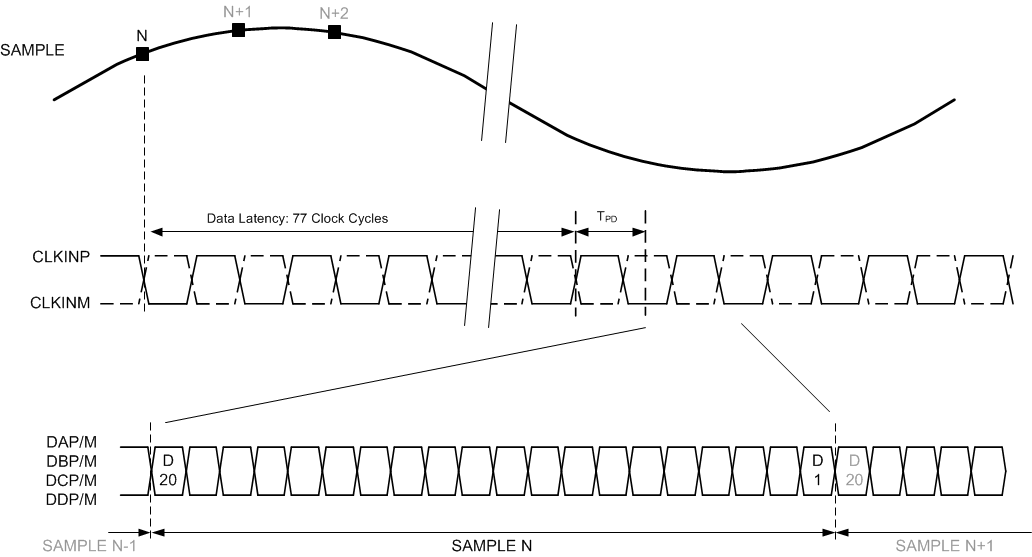Figure 1. Latency Timing Diagram

### 6.9 Typical Characteristics: 14-Bit Burst Mode

Typical values are at TA = 25°C, full temperature range is from TMIN = –40°C to TMAX = 85°C, ADC Sampling Frequency = 500 Msps, 14-bit Resolution, No Decimation Filter, 50% clock duty cycle, AVDD3V = 3 V, AVDD = DVDD = 1.9 V, IOVDD = 1.15 V, –1-dBFS differential input for IF ≤ 250 MHz, and –3-dBFS differential input for IF > 250 MHz, unless otherwise noted.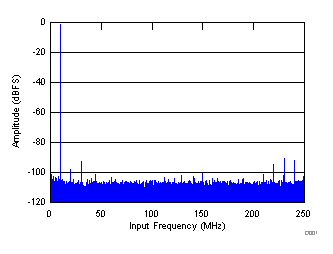FIN = 10 MHz , AIN = –1 dBFS SNR = 71 dBFS, SFDR = 89 dBc, SFDR = 89 dBc (Non23)
Figure 2. FFT for 10-MHz Input SignalFIN = 190 MHz , AIN = –1 dBFS SNR = 69.4 dBFS, SFDR = 88 dBc, SFDR = 96 dBc (Non23)
Figure 4. FFT for 190-MHz Input Signal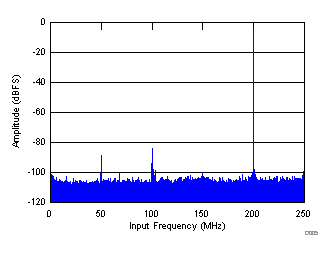FIN = 300 MHz , AIN = - 3 dBFS SNR = 69.4 dBFS, SFDR = 80 dBc, SFDR = 95 dBc (Non23)
Figure 6. FFT for 300-MHz Input SignalFIN = 470 MHz , AIN = - 3 dBFS SNR = 67.4 dBFS, SFDR = 73 dBc, SFDR = 80 dBc (Non23)
Figure 8. FFT for 470-MHz Input SignalFIN1 = 185 MHz, FIN2 = 190 MHz, IMD = 103 dBFS Each tone at -36 dBFS
Figure 10. FFT for Two-Tone Input Signal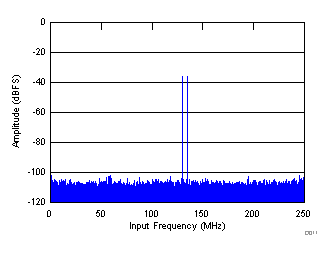FIN1 = 370 MHz, FIN2 = 365 MHz, IMD = 102 dBFS Each tone at -36 dBFS
Figure 12. FFT for Two-Tone Input Signal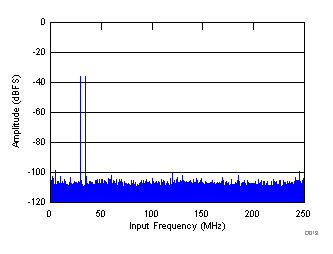FIN1 = 470 MHz, FIN2 = 465 MHz, IMD = 98.8 dBFS Each tone at -36 dBFS
Figure 14. FFT for Two-Tone Input Signal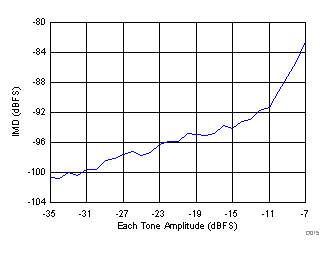FIN1 = 365 MHz, FIN2 = 370 MHz
Figure 16. Intermodulation Distortion Vs Input Amplitude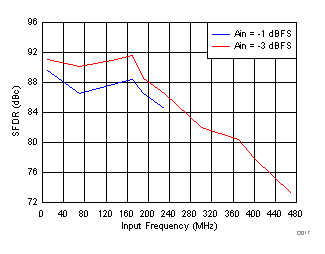Figure 18. Spurious-Free Dynamic Range vs Input Frequency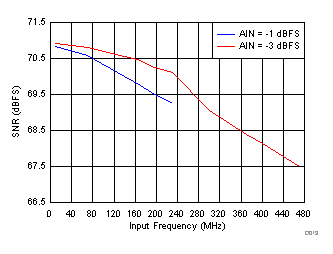Figure 20. Signal-to-Noise Ratio vs Input Frequency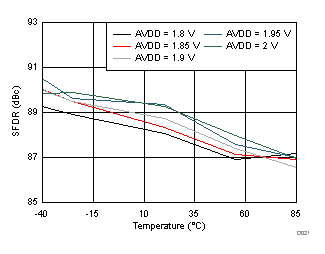FIN = 190 MHz, AIN = – 1 dBFS
Figure 22. Spurious-Free Dynamic Range vs AVDD Supply and Temperature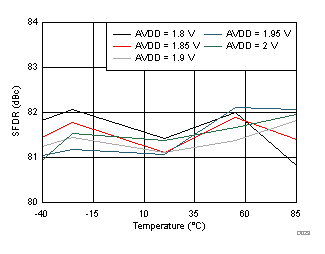FIN = 370 MHz, AIN = – 3 dBFS
Figure 24. Spurious-Free Dynamic Range vs AVDD Supply and Temperature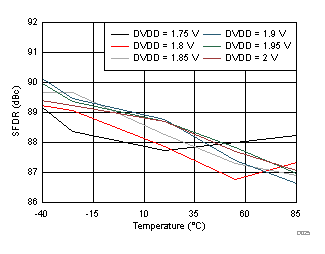FIN = 190 MHz, AIN = – 1 dBFS
Figure 26. Spurious-Free Dynamic Range vs DVDD Supply and Temperature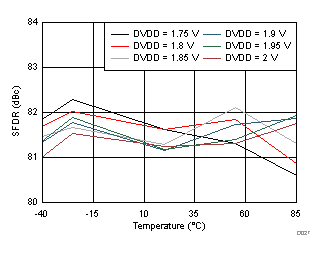FIN = 370 MHz, AIN = – 3 dBFS
Figure 28. Spurious-Free Dynamic Range vs DVDD Supply and Temperature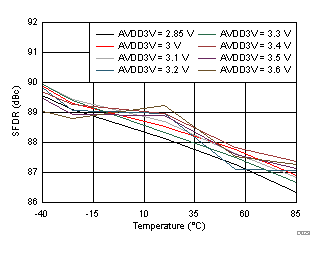FIN = 190 MHz, AIN = – 1 dBFS
Figure 30. Spurious-Free Dynamic Range vs AVDD3V Supply and Temperature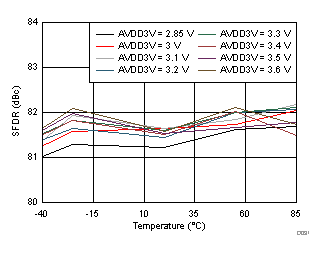FIN = 370 MHz, AIN = – 3 dBFS
Figure 32. Spurious-Free Dynamic Range vs AVDD3V Supply and TemperatureFIN = 370 MHz
Figure 34. Performance vs AmplitudeFIN = 370 MHz, AIN = – 3 dBFS
Figure 36. Performance vs Clock Amplitude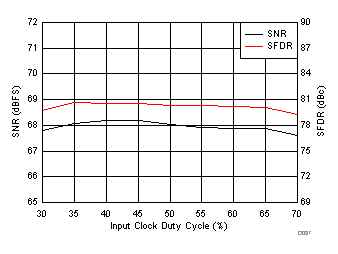FIN = 370 MHz, AIN = – 3 dBFS
Figure 38. Performance vs Clock Duty Cycle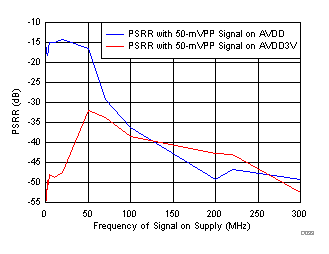FIN = 190 MHz, AIN = – 1 dBFS
Figure 40. Power-Supply Rejection Ratio vs Supplies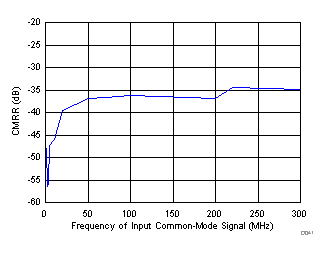FIN = 190 MHz, AIN= – 1dBFS 50-mVPP test-Signal on input common mode
Figure 42. Common-Mode Rejection Ratio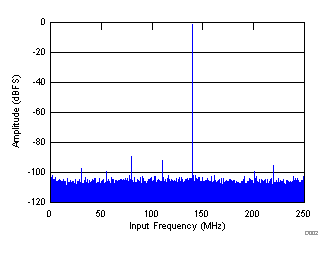FIN = 140 MHz , AIN = –1 dBFS SNR = 70 dBFS, SFDR = 88 dBc, SFDR = 91 dBc (Non23)
Figure 3. FFT for 140-MHz Input Signal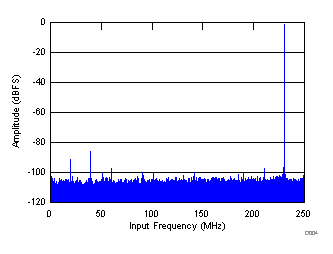FIN = 230 MHz , AIN = –1 dBFS SNR = 69.4 dBFS, SFDR = 85 dBc, SFDR = 96 dBc (Non23)
Figure 5. FFT for 230-MHz Input Signal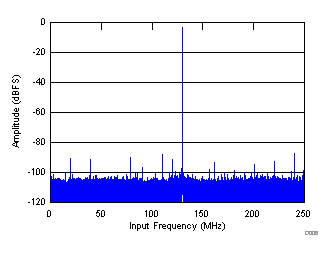FIN = 370 MHz , AIN = - 3 dBFS SNR = 68.4 dBFS, SFDR = 84 dBc, SFDR = 86 dBc (Non23)
Figure 7. FFT for 370-MHz Input Signal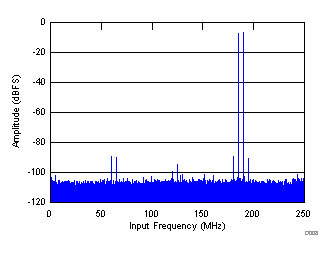FIN1 = 185 MHz, FIN2 = 190 MHz, IMD = 89 dBFS Each tone at -7 dBFS
Figure 9. FFT for Two-Tone Input Signal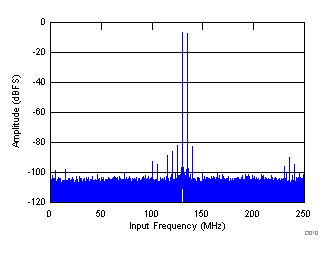FIN1 = 370 MHz, FIN2 = 365 MHz, IMD = 81.7 dBFS Each tone at -7 dBFS
Figure 11. FFT for Two-Tone Input Signal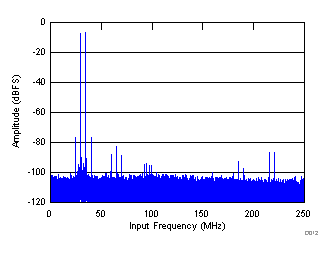FIN1 = 470 MHz, FIN2 = 465 MHz, IMD = 76.7 dBFS Each tone at -7 dBFS
Figure 13. FFT for Two-Tone Input Signal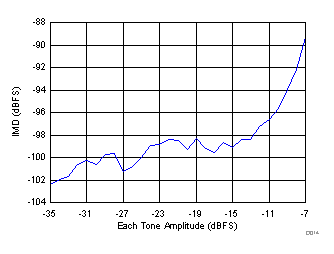FIN1 = 185 MHz, FIN2 = 190 MHz
Figure 15. Intermodulation Distortion Vs Input Amplitude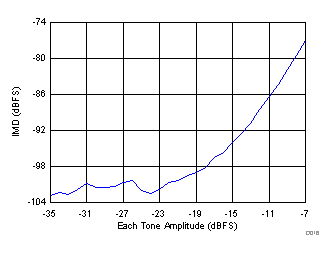FIN1 = 465 MHz, FIN2 = 470 MHz
Figure 17. Intermodulation Distortion Vs Input Amplitude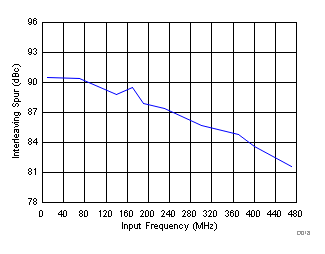Figure 19. IL Spur Vs Input Frequency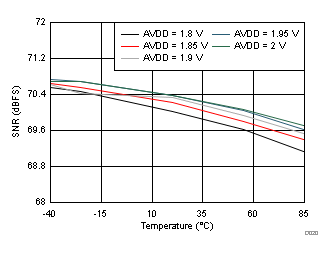FIN = 190 MHz, AIN = – 1 dBFS
Figure 21. Signal-to-Noise Ratio vs AVDD Supply and TemperatureFIN = 370 MHz, AIN = – 3 dBFS
Figure 23. Signal-to-Noise Ratio vs AVDD Supply and Temperature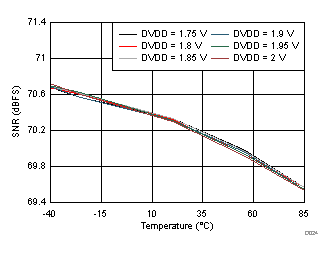FIN = 190 MHz, AIN = – 1 dBFS
Figure 25. Signal-to-Noise Ratio vs DVDD Supply and Temperature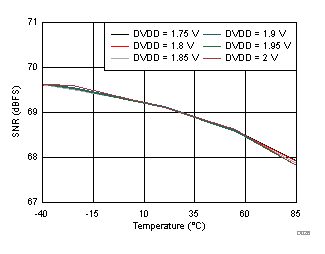FIN = 370 MHz, AIN = – 3 dBFS
Figure 27. Signal-to-Noise Ratio vs DVDD Supply and Temperature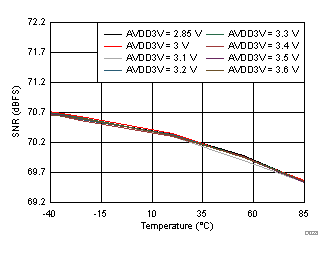FIN = 190 MHz, AIN = – 1 dBFS
Figure 29. Signal-to-Noise Ratio vs AVDD3V Supply and Temperature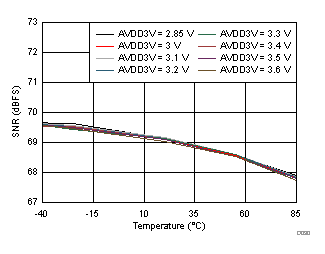FIN = 370 MHz, AIN = – 3 dBFS
Figure 31. Signal-to-Noise Ratio vs AVDD3V Supply and TemperatureFIN = 190 MHz
Figure 33. Performance vs Amplitude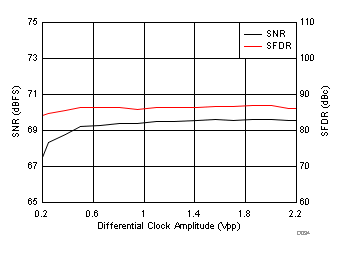FIN = 190 MHz, AIN = – 1 dBFS
Figure 35. Performance vs Clock Amplitude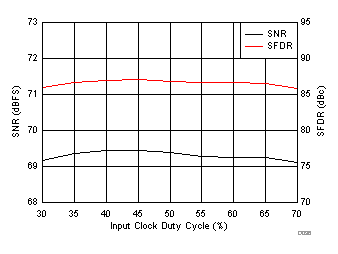FIN = 190 MHz, AIN = – 1 dBFS
Figure 37. Performance vs Clock Duty Cycle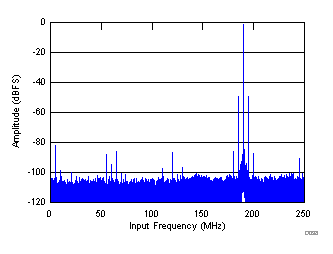FIN = 190 MHz , AIN = –1 dBFS SFDR = 49 dBc, fPSRR = 5 MHz, APSRR = 50 mVPP
Figure 39. Power-Supply Rejection Ratio FFT for test signal on AVDD Supply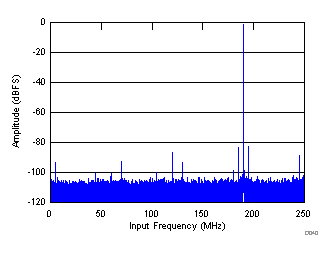FIN = 190 MHz , AIN = – 1 dBFS SFDR = 81 , fCMRR = 5 MHz, ACMRR = 50 mVPP
Figure 41. Common-Mode Rejection Ratio FFT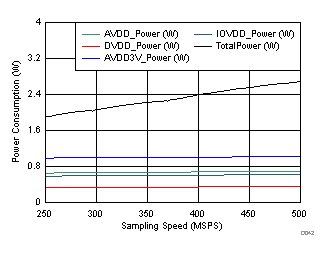Figure 43. Power vs Chip Clock

### 6.10 Typical Characteristics: Mode 2

Low pass or high pass decimation-by-2 filter selected as per input frequency. Typical values are at TA = 25°C, full temperature range is from TMIN = –40°C to TMAX = 85°C, ADC Sampling Frequency = 500 Msps, 14-bit Resolution, No Decimation Filter, 50% clock duty cycle, AVDD3V = 3 V, AVDD = DVDD = 1.9 V, IOVDD = 1.15 V, –1-dBFS differential input for IF ≤ 250 MHz, and –3-dBFS differential input for IF > 250 MHz, unless otherwise noted.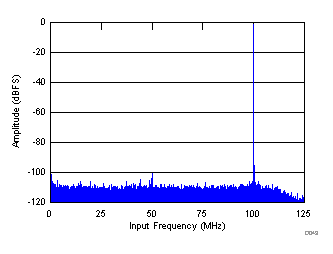FIN = 100 MHz , AIN = – 1 dBFS SNR = 74.1 dBFS, SFDR = 98 dBc, SFDR = 100 dBc (Non23)
Figure 44. FFT for 100-MHz Input Signal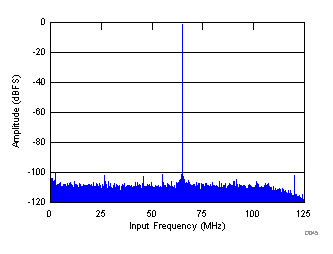FIN = 185 MHz , AIN = – 1 dBFS SNR = 73.2 dBFS, SFDR = 98 dBc, SFDR = 98 dBc (Non23)
Figure 46. FFT for 185-MHz Input Signal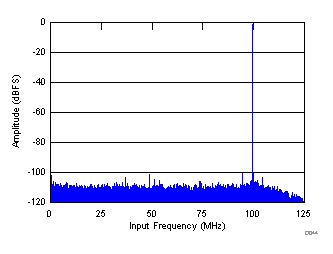FIN = 150 MHz , AIN = – 1 dBFS SNR = 73.8 dBFS, SFDR = 99 dBc, SFDR = 99 dBc (Non23)
Figure 45. FFT for 150-MHz Input Signal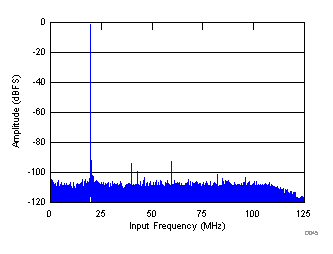FIN = 230 MHz , AIN = – 1 dBFS SNR = 72.4 dBFS, SFDR = 91 dBc, SFDR = 98 dBc (Non23)
Figure 47. FFT for 230-MHz Input Signal

### 6.11 Typical Characteristics: Mode 0

Low-pass decimation-by-2 filter selected, Complex FFT plotted,mixer frequency 125 MHz. Typical values are at TA = 25°C, full temperature range is from TMIN = –40°C to TMAX = 85°C, ADC Sampling Frequency = 500 Msps, 14-bit Resolution, No Decimation Filter, 50% clock duty cycle, AVDD3V = 3 V, AVDD = DVDD = 1.9 V, IOVDD = 1.15 V, –1-dBFS differential input for IF ≤ 250 MHz, and –3-dBFS differential input for IF > 250 MHz, unless otherwise noted.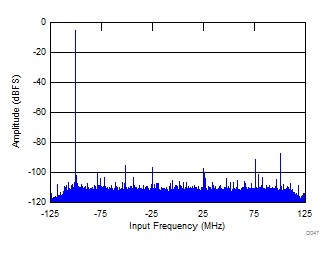FIN = 270 MHz , AIN = – 3 dBFS SNR = 69.5 dBFS, SFDR = 83 dBc, SFDR = 87 dBc (Non23)
Figure 48. FFT for 270-MHz Input Signal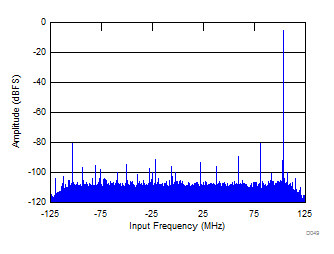FIN = 470 MHz , AIN = – 3 dBFS SNR = 66.3 dBFS, SFDR = 75 dBc, SFDR = 75 dBc (Non23)
Figure 50. FFT for 470-MHz Input Signal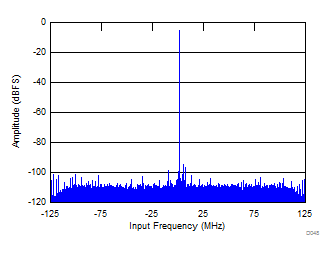FIN = 370 MHz , AIN = – 3 dBFS SNR = 68.1 dBFS, SFDR = 82 dBc, SFDR = 82 dBc (Non23)
Figure 49. FFT for 370-MHz Input Signal